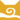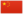—— 白尾，第四学徒》，第126页

Whitetail风族 猫后风族 武士风族 猫后风族 武士风族 长老白爪 Whitepaw  白掌
 白尾 Whitetail  白尾

Q2449：风族母猫，自《预言开始》系列登场的角色

## 细节

### 外貌

• 她是一只身形娇小的白色母猫。:猫物表
• Su[?]认为她有着绿色的眼睛。

### 勘误

• 她曾被错误地称呼为白翅。:14
• 她曾被错误地描述为影族猫。:104

## 语录

—— 狮焰向鸽爪介绍白尾，《第四学徒》，第115页

—— 白尾对蟾足，《第四学徒》，第123页

—— 狮焰对白尾的印象，《第四学徒》，第130页

—— 白尾警告鸽爪和常春藤爪，《战声渐近》，第64页

## 登场书目

• 寒冰烈火 (Q59)[配角][首次登场🐱]
• 疑云重重 (Q60)[猫物闲角]
• 风起云涌 (Q65)[猫物闲角]
• 险路惊魂 (Q66)[猫物闲角]
• 力挽狂澜 (Q67)[猫物闲角]
• 午夜追踪 (Q139)[猫物闲角]
• 新月危机 (Q140)[猫物闲角]
• 重现家园 (Q141)[猫物闲角]
• 星光指路 (Q142)[配角]
• 黄昏战争 (Q143)[配角]
• 日落和平 (Q144)[猫物闲角]
• 预视力量 (Q152)[配角]
• 暗河汹涌 (Q153)[配角]
• 驱逐之战 (Q154)[配角]
• 天蚀遮月 (Q155)[猫物闲角]
• 暗夜长影 (Q156)[猫物闲角]
• 拂晓之光 (Q157)[配角]
• 第四学徒 (Q158)[配角]
• 战声渐近 (Q159)[配角]
• 暗夜密语 (Q160)[对话提及]
• 月光印记 (Q161)[猫物闲角]
• 武士归来 (Q162)[配角]
• 群星之战 (Q163)[配角]
• 学徒探索 (Q170)[猫物闲角]
• 雷影交加 (Q171)[猫物闲角]
• 天空破碎 (Q172)[配角]
• 极夜无光 (Q173)[猫物闲角]
• 烈焰焚河 (Q174)[配角]
• 风暴来袭 (Q175)[猫物闲角]
• 火星的探索 (Q176)[猫物闲角]
• 黑莓星的风暴 (Q182)[猫物闲角]
• 虎心的阴影 (Q185)[猫物闲角]
• 鸦羽的拷问 (Q186)[配角]
• 松鼠飞的希冀 (Q3561)[对话提及][死亡时刻✝]
• 灰条的誓言 (Q4057)[猫物闲角]
• 冬青叶的故事 (Q187)[猫物闲角]
• 雾星的征兆 (Q188)[猫物闲角]
• 虎掌的愤怒 (Q190)[猫物闲角]
• 叶池的祈愿 (Q191)[猫物闲角]
• 鸽翅的沉默 (Q192)[猫物闲角]
• 褐皮的族群 (Q3566)[猫物闲角]
• 蛾翅的秘密 (Q3628)[猫物闲角]
• 黑脚的清算 (Q4336)[猫物闲角]
• 斑毛的叛变 (Q4337)[猫物闲角]
• 族群的秘密 (Q199)[对话提及][追溯修订]
• 族群的战争 (Q202)[配角]
• 超越守则：光灵的恻隐之心 (Q4321)[配角]

## 参考文献

这篇文章基于CC BY-SA 3.0许可使用了猫武士维基（英语）Whitetail (WC)一文中的部分内容。
1. 《松鼠飞的希望》第一章 (Q3799)
2. 寒冰烈火 (Q59)
3. Official Website (2019-01-07). Family Tree. WarriorCats.com.
4. 寒冰烈火 (Q59)险路惊魂 (Q66)
5. 险路惊魂 (Q66)午夜追踪 (Q139)
6. 午夜追踪 (Q139)重现家园 (Q141)
7. 重现家园 (Q141)预视力量 (Q152)
8. 预视力量 (Q152)鸽翅的沉默 (Q192)
9. 险路惊魂 (Q66)
10. 预视力量 (Q152)
11. 预视力量 (Q152)暗夜长影 (Q156)
12. 险路惊魂
13. Su Susann (2017-04-20). 站内截图. Facebook. 原页面 归档于 2017-11-02.
14. Su Susann (2017-04-24). 站内截图. Facebook. 原页面 归档于 2017-11-02.
15. Su Susann (2017-04-26). 站内截图. Facebook. 原页面 归档于 2017-11-02.
16. 星光指路
17. 族群的战争
0.0
0人评价